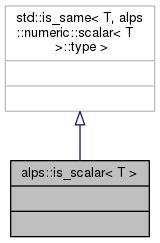ALPSCore reference
alps::is_scalar< T > Struct Template Reference

Metafunction-predicate: returns true_type if type T is scalar. More...

#include <is_scalar.hpp>

Inheritance diagram for alps::is_scalar< T >:Collaboration diagram for alps::is_scalar< T >:Detailed Description

template<typename T> struct alps::is_scalar< T >

Metafunction-predicate: returns true_type if type T is scalar.

Parameters
 T type to test
Returns
std::true_type or std::false_type

The type T is scalar iff it's "mathematical scalar" (as defined by metafunction alps::numeric::scalar<T>) is the same as T.

Definition at line 24 of file is_scalar.hpp.

The documentation for this struct was generated from the following file: